Скачать презентацию Additive Rule Contingency Table Experiment Draw 1 card

fb90a816531016bcc645094225f395fd.ppt

• Количество слайдов: 19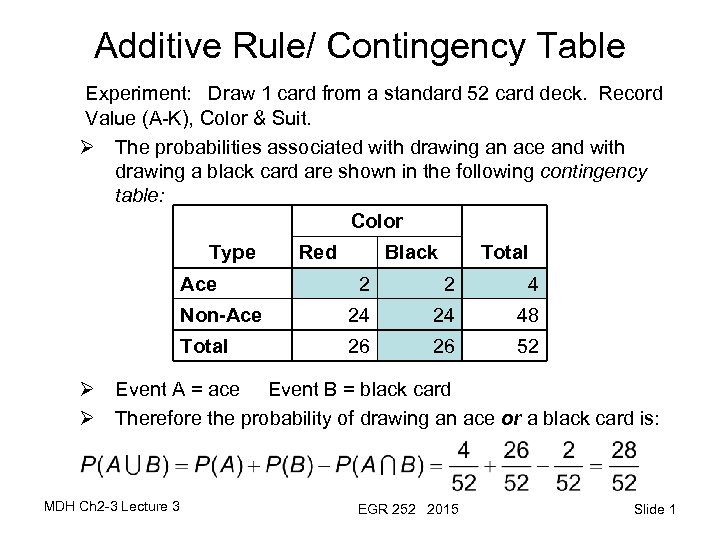Additive Rule/ Contingency Table Experiment: Draw 1 card from a standard 52 card deck. Record Value (A-K), Color & Suit. Ø The probabilities associated with drawing an ace and with drawing a black card are shown in the following contingency table: Color Type Ace Red Black Total 2 2 4 Non-Ace 24 24 48 Total 26 26 52 Ø Event A = ace Event B = black card Ø Therefore the probability of drawing an ace or a black card is: MDH Ch 2 -3 Lecture 3 EGR 252 2015 Slide 1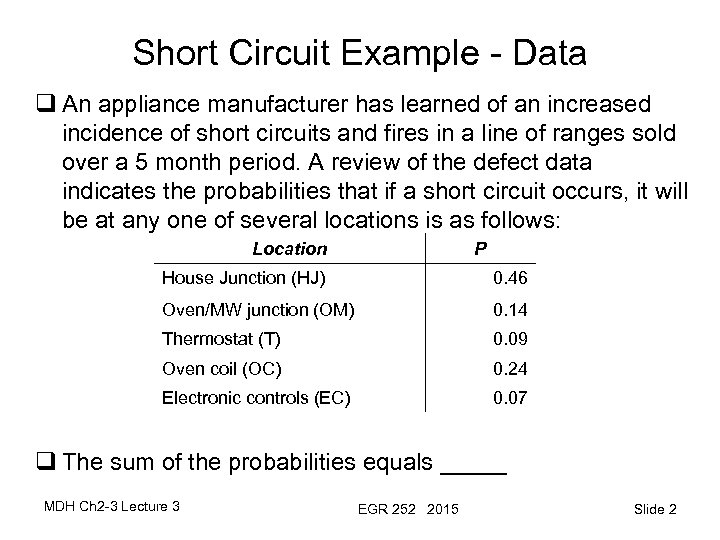Short Circuit Example - Data q An appliance manufacturer has learned of an increased incidence of short circuits and fires in a line of ranges sold over a 5 month period. A review of the defect data indicates the probabilities that if a short circuit occurs, it will be at any one of several locations is as follows: Location P House Junction (HJ) 0. 46 Oven/MW junction (OM) 0. 14 Thermostat (T) 0. 09 Oven coil (OC) 0. 24 Electronic controls (EC) 0. 07 q The sum of the probabilities equals _____ MDH Ch 2 -3 Lecture 3 EGR 252 2015 Slide 2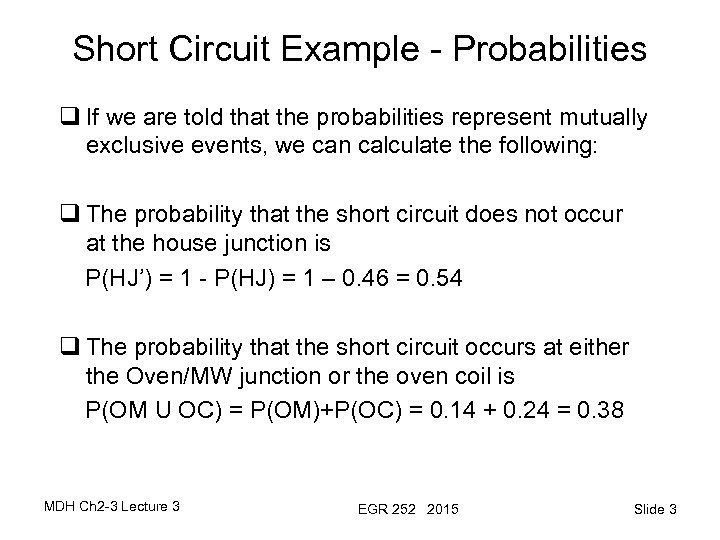Short Circuit Example - Probabilities q If we are told that the probabilities represent mutually exclusive events, we can calculate the following: q The probability that the short circuit does not occur at the house junction is P(HJ’) = 1 - P(HJ) = 1 – 0. 46 = 0. 54 q The probability that the short circuit occurs at either the Oven/MW junction or the oven coil is P(OM U OC) = P(OM)+P(OC) = 0. 14 + 0. 24 = 0. 38 MDH Ch 2 -3 Lecture 3 EGR 252 2015 Slide 3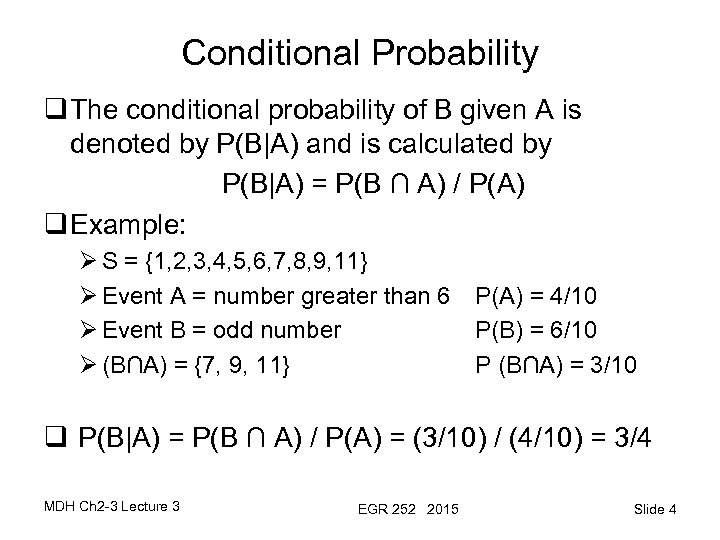Conditional Probability q The conditional probability of B given A is denoted by P(B|A) and is calculated by P(B|A) = P(B ∩ A) / P(A) q Example: Ø S = {1, 2, 3, 4, 5, 6, 7, 8, 9, 11} Ø Event A = number greater than 6 Ø Event B = odd number Ø (B∩A) = {7, 9, 11} P(A) = 4/10 P(B) = 6/10 P (B∩A) = 3/10 q P(B|A) = P(B ∩ A) / P(A) = (3/10) / (4/10) = 3/4 MDH Ch 2 -3 Lecture 3 EGR 252 2015 Slide 4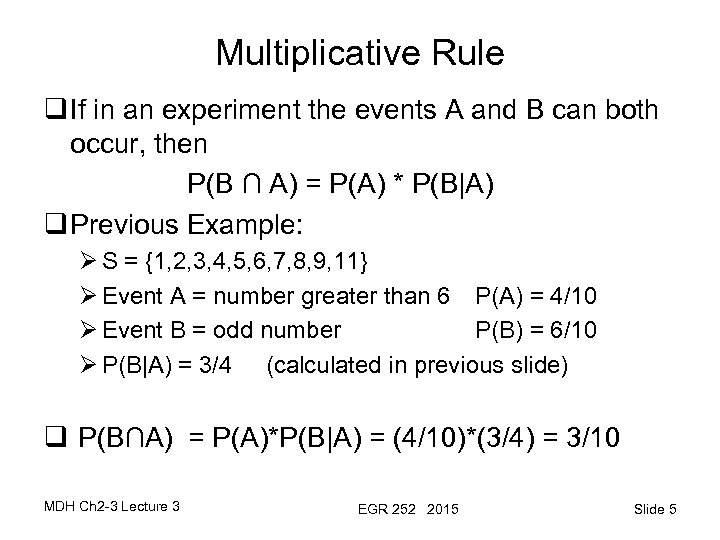Multiplicative Rule q If in an experiment the events A and B can both occur, then P(B ∩ A) = P(A) * P(B|A) q Previous Example: Ø S = {1, 2, 3, 4, 5, 6, 7, 8, 9, 11} Ø Event A = number greater than 6 P(A) = 4/10 Ø Event B = odd number P(B) = 6/10 Ø P(B|A) = 3/4 (calculated in previous slide) q P(B∩A) = P(A)*P(B|A) = (4/10)*(3/4) = 3/10 MDH Ch 2 -3 Lecture 3 EGR 252 2015 Slide 5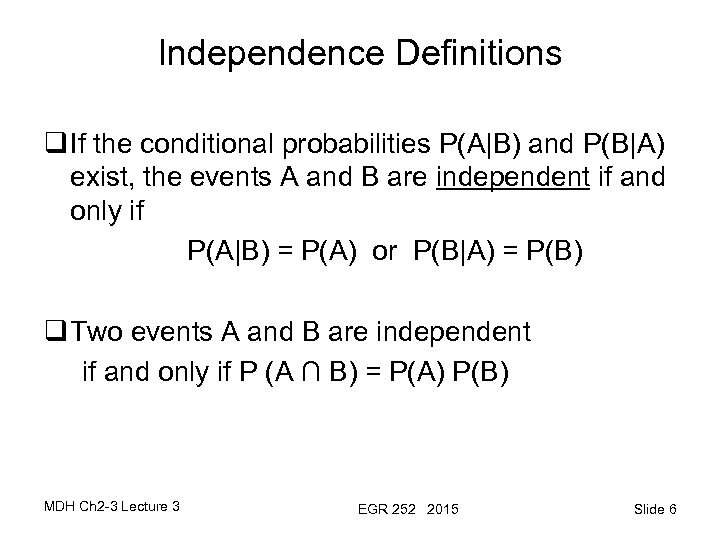Independence Definitions q If the conditional probabilities P(A|B) and P(B|A) exist, the events A and B are independent if and only if P(A|B) = P(A) or P(B|A) = P(B) q Two events A and B are independent if and only if P (A ∩ B) = P(A) P(B) MDH Ch 2 -3 Lecture 3 EGR 252 2015 Slide 6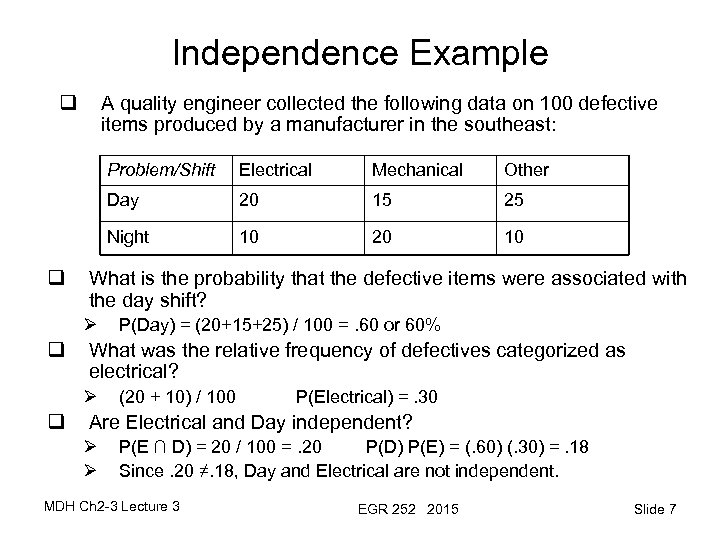Independence Example q A quality engineer collected the following data on 100 defective items produced by a manufacturer in the southeast: Problem/Shift 20 15 25 10 20 10 What is the probability that the defective items were associated with the day shift? Ø P(Day) = (20+15+25) / 100 =. 60 or 60% What was the relative frequency of defectives categorized as electrical? Ø q Other Night q Mechanical Day q Electrical (20 + 10) / 100 P(Electrical) =. 30 Are Electrical and Day independent? Ø Ø P(E ∩ D) = 20 / 100 =. 20 P(D) P(E) = (. 60) (. 30) =. 18 Since. 20 ≠. 18, Day and Electrical are not independent. MDH Ch 2 -3 Lecture 3 EGR 252 2015 Slide 7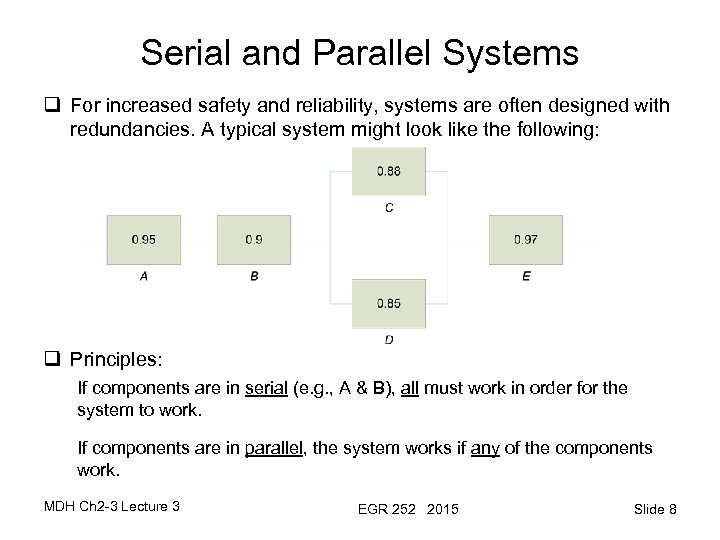Serial and Parallel Systems q For increased safety and reliability, systems are often designed with redundancies. A typical system might look like the following: q Principles: If components are in serial (e. g. , A & B), all must work in order for the system to work. If components are in parallel, the system works if any of the components work. MDH Ch 2 -3 Lecture 3 EGR 252 2015 Slide 8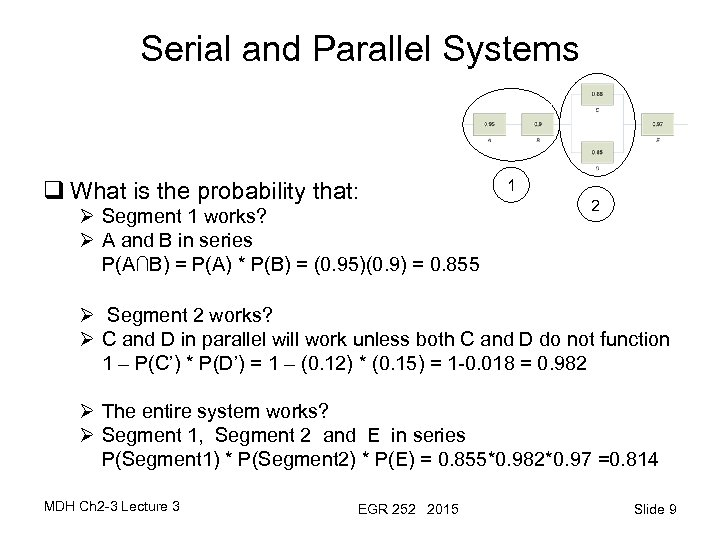Serial and Parallel Systems q What is the probability that: Ø Segment 1 works? Ø A and B in series P(A∩B) = P(A) * P(B) = (0. 95)(0. 9) = 0. 855 1 2 Ø Segment 2 works? Ø C and D in parallel will work unless both C and D do not function 1 – P(C’) * P(D’) = 1 – (0. 12) * (0. 15) = 1 -0. 018 = 0. 982 Ø The entire system works? Ø Segment 1, Segment 2 and E in series P(Segment 1) * P(Segment 2) * P(E) = 0. 855*0. 982*0. 97 =0. 814 MDH Ch 2 -3 Lecture 3 EGR 252 2015 Slide 9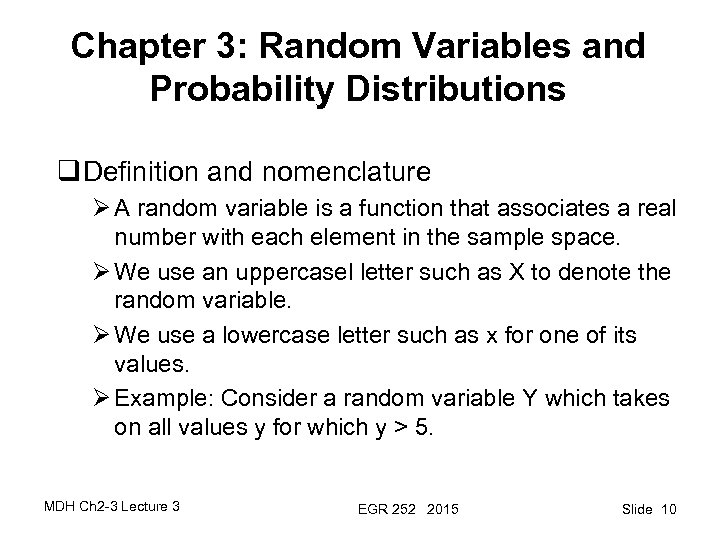Chapter 3: Random Variables and Probability Distributions q Definition and nomenclature Ø A random variable is a function that associates a real number with each element in the sample space. Ø We use an uppercasel letter such as X to denote the random variable. Ø We use a lowercase letter such as x for one of its values. Ø Example: Consider a random variable Y which takes on all values y for which y > 5. MDH Ch 2 -3 Lecture 3 EGR 252 2015 Slide 10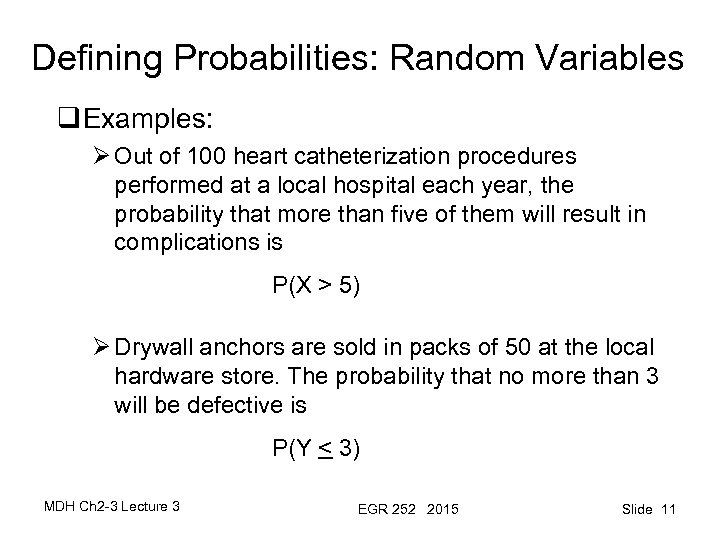Defining Probabilities: Random Variables q Examples: Ø Out of 100 heart catheterization procedures performed at a local hospital each year, the probability that more than five of them will result in complications is P(X > 5) Ø Drywall anchors are sold in packs of 50 at the local hardware store. The probability that no more than 3 will be defective is P(Y < 3) MDH Ch 2 -3 Lecture 3 EGR 252 2015 Slide 11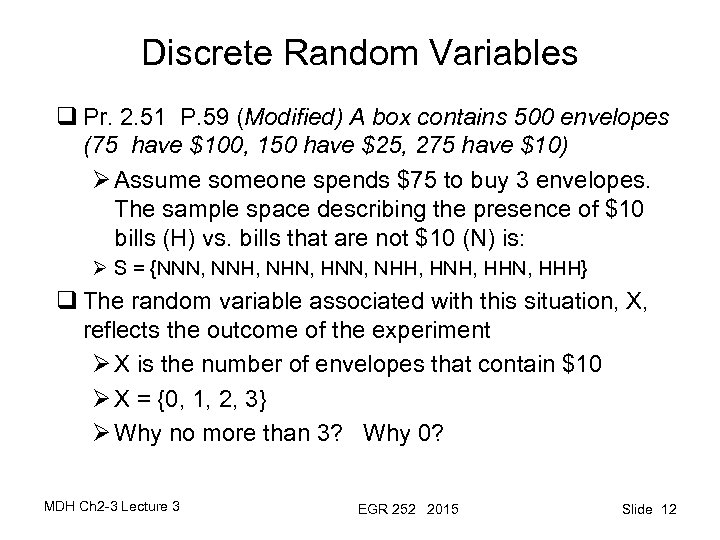Discrete Random Variables q Pr. 2. 51 P. 59 (Modified) A box contains 500 envelopes (75 have \$100, 150 have \$25, 275 have \$10) Ø Assume someone spends \$75 to buy 3 envelopes. The sample space describing the presence of \$10 bills (H) vs. bills that are not \$10 (N) is: Ø S = {NNN, NNH, NHN, HNN, NHH, HNH, HHN, HHH} q The random variable associated with this situation, X, reflects the outcome of the experiment Ø X is the number of envelopes that contain \$10 Ø X = {0, 1, 2, 3} Ø Why no more than 3? Why 0? MDH Ch 2 -3 Lecture 3 EGR 252 2015 Slide 12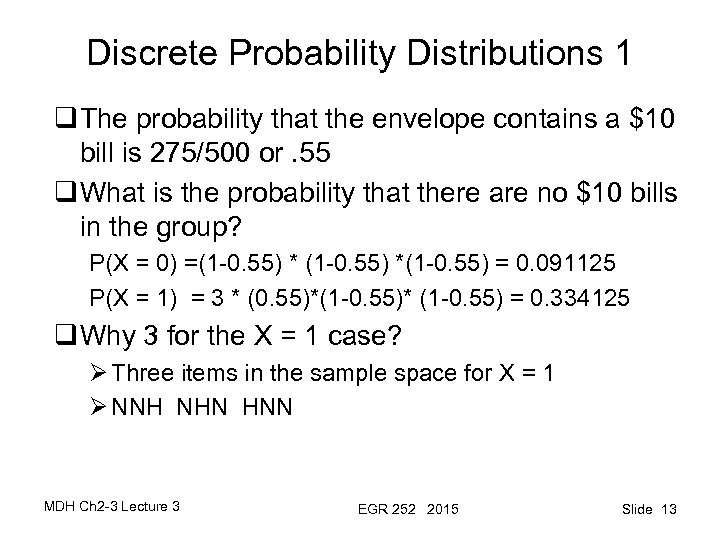Discrete Probability Distributions 1 q The probability that the envelope contains a \$10 bill is 275/500 or. 55 q What is the probability that there are no \$10 bills in the group? P(X = 0) =(1 -0. 55) *(1 -0. 55) = 0. 091125 P(X = 1) = 3 * (0. 55)*(1 -0. 55)* (1 -0. 55) = 0. 334125 q Why 3 for the X = 1 case? Ø Three items in the sample space for X = 1 Ø NNH NHN HNN MDH Ch 2 -3 Lecture 3 EGR 252 2015 Slide 13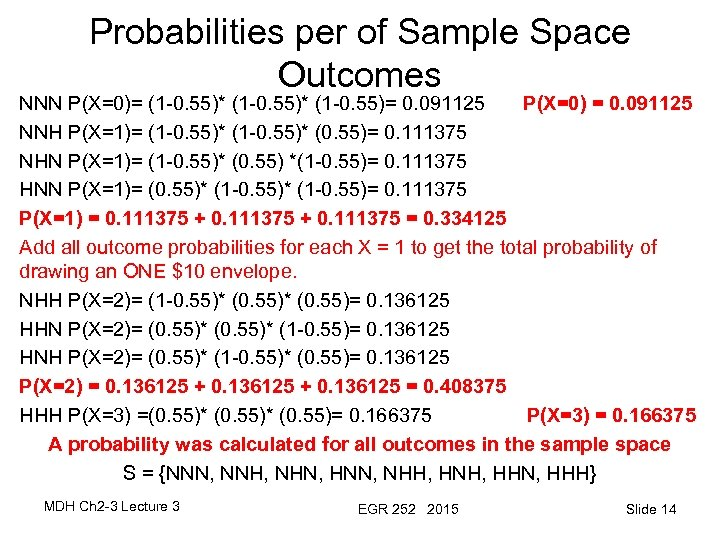Probabilities per of Sample Space Outcomes NNN P(X=0)= (1 -0. 55)* (1 -0. 55)= 0. 091125 P(X=0) = 0. 091125 NNH P(X=1)= (1 -0. 55)* (0. 55)= 0. 111375 NHN P(X=1)= (1 -0. 55)* (0. 55) *(1 -0. 55)= 0. 111375 HNN P(X=1)= (0. 55)* (1 -0. 55)= 0. 111375 P(X=1) = 0. 111375 + 0. 111375 = 0. 334125 Add all outcome probabilities for each X = 1 to get the total probability of drawing an ONE \$10 envelope. NHH P(X=2)= (1 -0. 55)* (0. 55)= 0. 136125 HHN P(X=2)= (0. 55)* (1 -0. 55)= 0. 136125 HNH P(X=2)= (0. 55)* (1 -0. 55)* (0. 55)= 0. 136125 P(X=2) = 0. 136125 + 0. 136125 = 0. 408375 HHH P(X=3) =(0. 55)* (0. 55)= 0. 166375 P(X=3) = 0. 166375 A probability was calculated for all outcomes in the sample space S = {NNN, NNH, NHN, HNN, NHH, HNH, HHN, HHH} MDH Ch 2 -3 Lecture 3 EGR 252 2015 Slide 14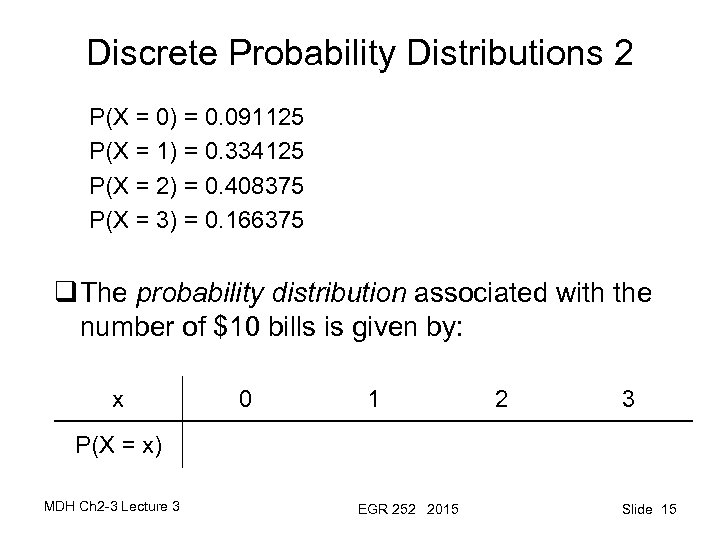Discrete Probability Distributions 2 P(X = 0) = 0. 091125 P(X = 1) = 0. 334125 P(X = 2) = 0. 408375 P(X = 3) = 0. 166375 q The probability distribution associated with the number of \$10 bills is given by: x 0 1 2 3 P(X = x) MDH Ch 2 -3 Lecture 3 EGR 252 2015 Slide 15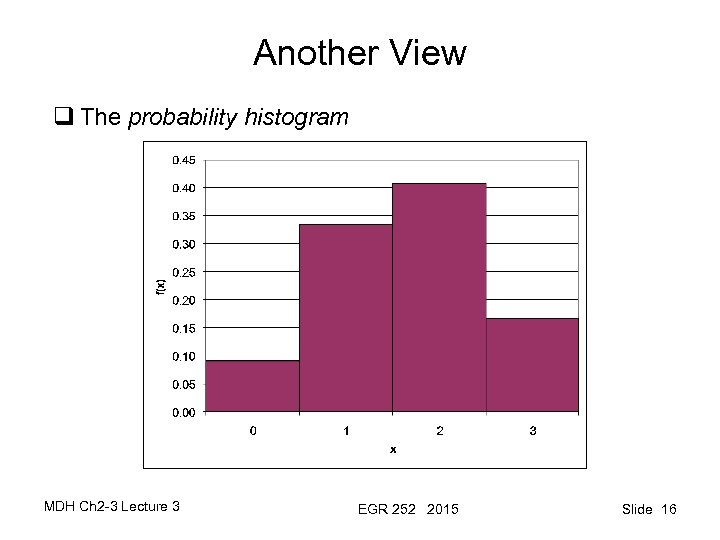Another View q The probability histogram MDH Ch 2 -3 Lecture 3 EGR 252 2015 Slide 16Another Discrete Probability Example Ø Given: § A shipment consists of 8 computers § 3 of the 8 are defective Ø Experiment: Randomly select 2 computers Ø Definition: random variable X = # of defective computers selected Ø What is the probability distribution for X? § Possible values for X: X = 0 X =1 X = 2 § Let’s start with P(X=0) [0 defectives and 2 nondefectives are selected] Recall that P = specified target / all possible (all ways to get 0 out of 3 defectives) ∩ (all ways to get 2 out of 5 nondefectives) (all ways to choose 2 out of 8 computers) MDH Ch 2 -3 Lecture 3 EGR 252 2015 Slide 17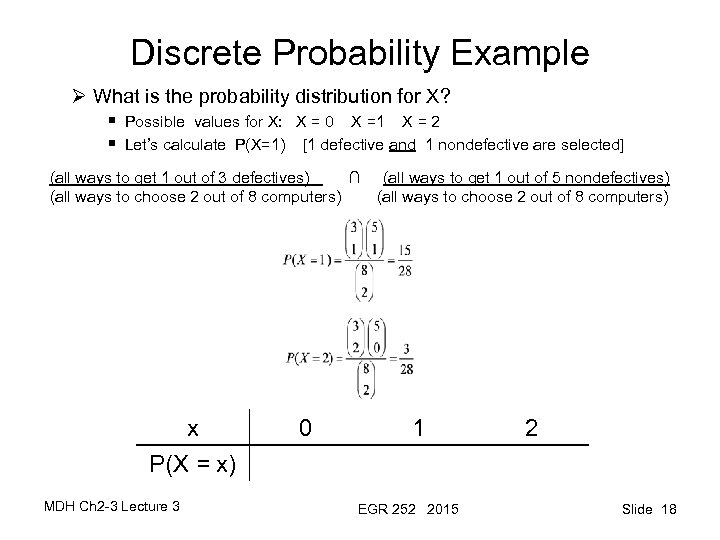Discrete Probability Example Ø What is the probability distribution for X? § Possible values for X: X = 0 X =1 X = 2 § Let’s calculate P(X=1) [1 defective and 1 nondefective are selected] (all ways to get 1 out of 3 defectives) ∩ (all ways to get 1 out of 5 nondefectives) (all ways to choose 2 out of 8 computers) x P(X = x) MDH Ch 2 -3 Lecture 3 0 1 EGR 252 2015 2 Slide 18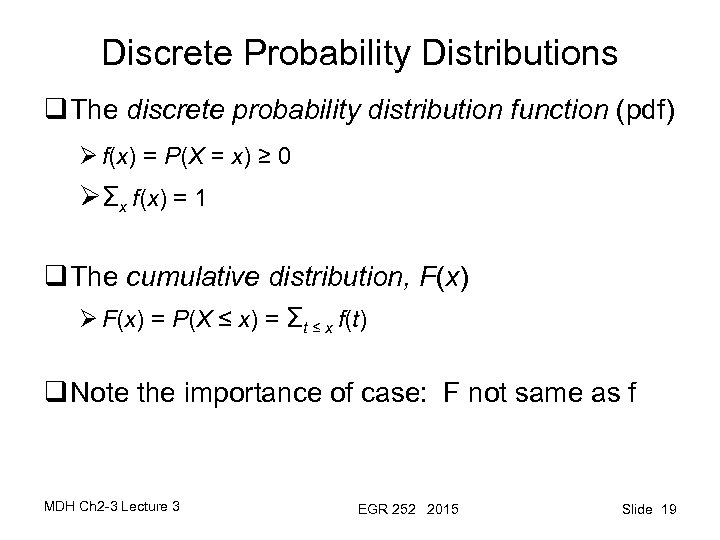Discrete Probability Distributions q The discrete probability distribution function (pdf) Ø f(x) = P(X = x) ≥ 0 ØΣx f(x) = 1 q The cumulative distribution, F(x) Ø F(x) = P(X ≤ x) = Σt ≤ x f(t) q Note the importance of case: F not same as f MDH Ch 2 -3 Lecture 3 EGR 252 2015 Slide 19